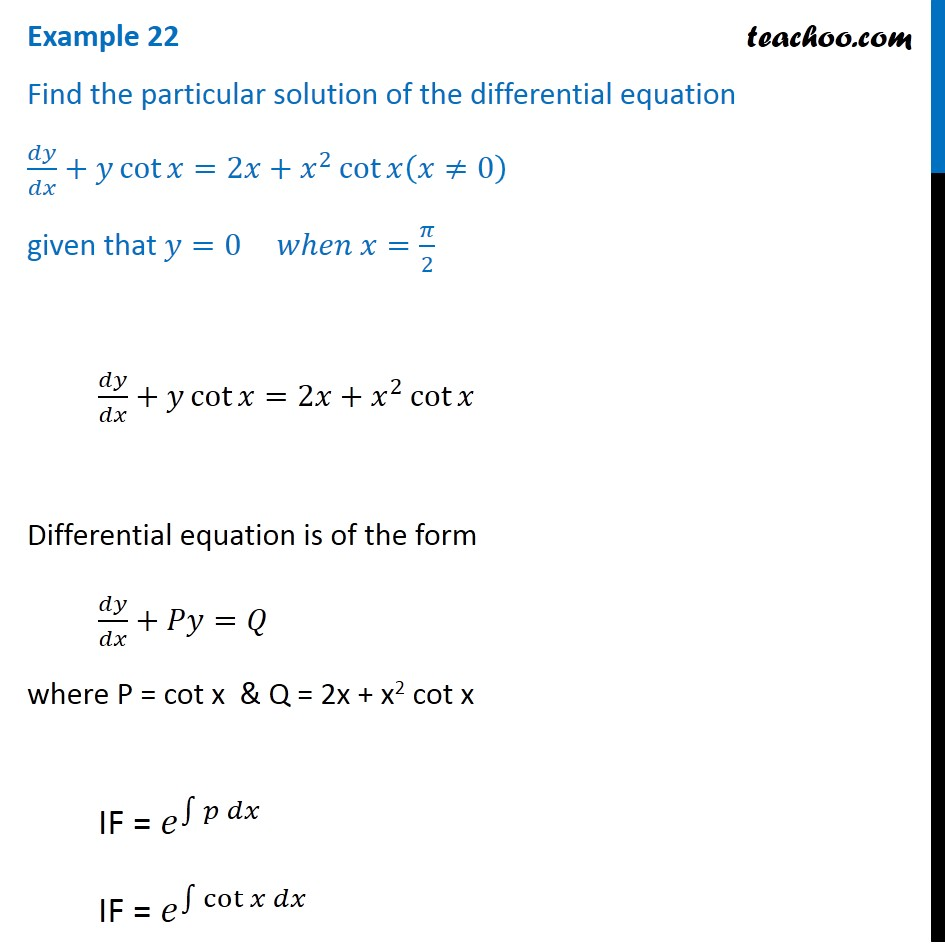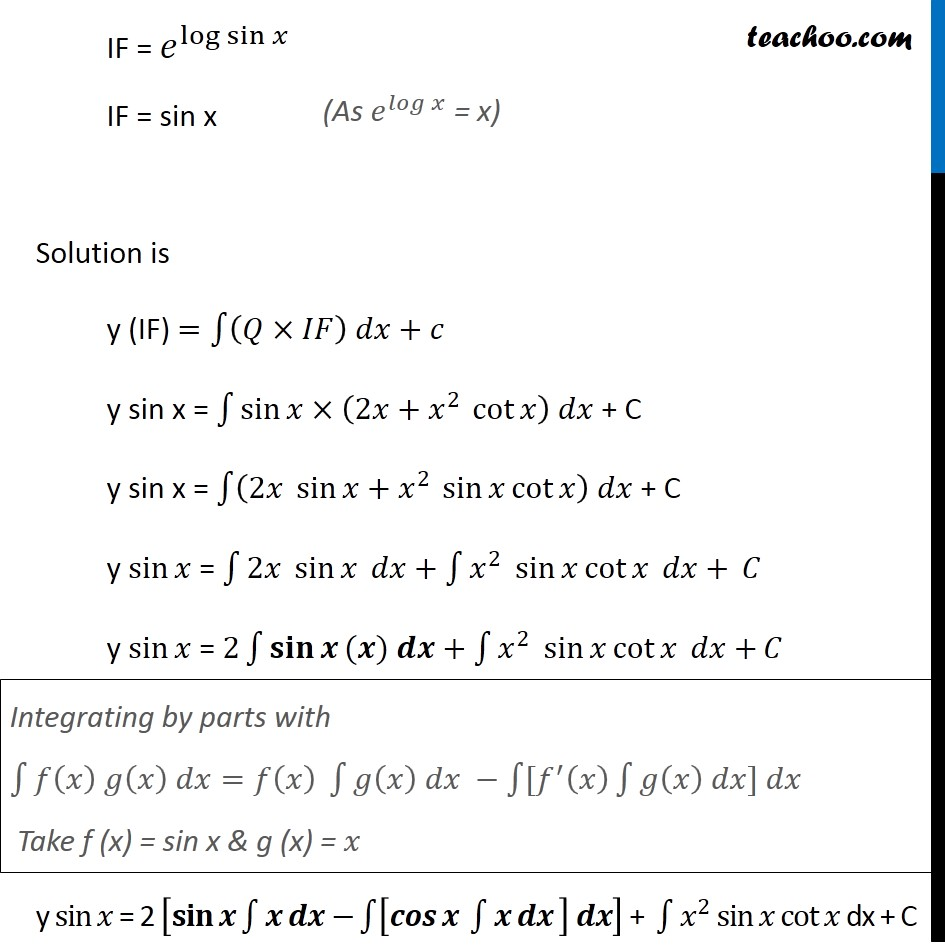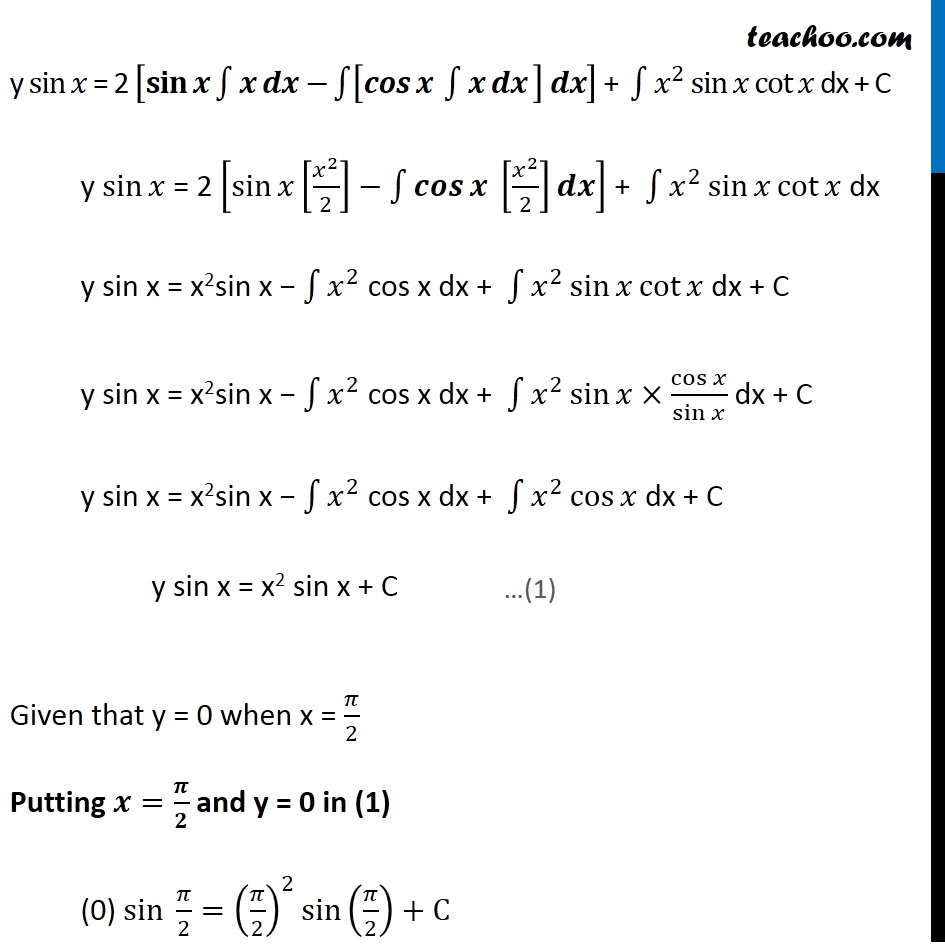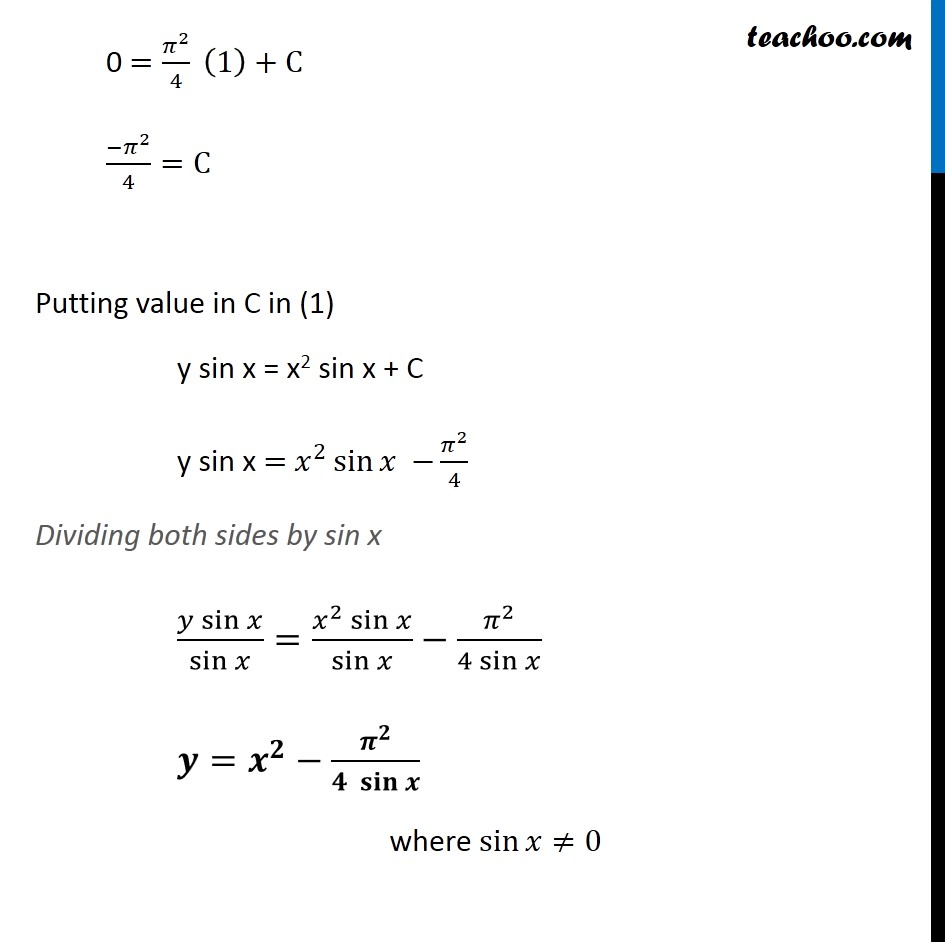1. Chapter 9 Class 12 Differential Equations
2. Serial order wise
3. Examples

Transcript

Example 22 Find the particular solution of the differential equation 𝑑𝑦/𝑑𝑥+𝑦 cot⁡〖𝑥=2𝑥+𝑥^2 cot⁡𝑥(𝑥≠0) 〗 given that 𝑦=0 𝑤ℎ𝑒𝑛 𝑥=𝜋/2 𝑑𝑦/𝑑𝑥+𝑦 cot⁡〖𝑥=2𝑥+𝑥^2 cot⁡𝑥 〗 Differential equation is of the form 𝑑𝑦/𝑑𝑥+𝑃𝑦=𝑄 where P = cot x & Q = 2x + x2 cot x IF = 𝑒^∫1▒〖𝑝 𝑑𝑥〗 IF = 𝑒^∫1▒〖cot⁡𝑥 𝑑𝑥〗 IF = 〖𝑒^log⁡sin⁡𝑥 〗^" " IF = sin x Solution is y (IF) =∫1▒〖(𝑄×𝐼𝐹) 𝑑𝑥+𝑐〗 y sin x = ∫1▒〖sin⁡𝑥×(2𝑥+𝑥^(2 ) cot⁡𝑥 ) 𝑑𝑥〗 + C y sin x = ∫1▒〖(2𝑥 sin⁡𝑥+𝑥^(2 ) sin⁡〖𝑥 cot⁡𝑥 〗 ) 𝑑𝑥〗 + C y sin⁡𝑥 = ∫1▒〖2𝑥 sin⁡𝑥 𝑑𝑥+〗 ∫1▒〖𝑥^2 sin⁡𝑥 cot⁡𝑥 𝑑𝑥+〗 𝐶 y sin⁡𝑥 = 2∫1▒〖𝐬𝐢𝐧⁡𝒙 (𝒙) 𝒅𝒙〗+∫1▒〖𝑥^2 sin⁡𝑥 cot⁡𝑥 𝑑𝑥+〗 𝐶 y sin⁡𝑥 = 2 [𝐬𝐢𝐧⁡𝒙 ∫1▒〖𝒙 𝒅𝒙−〗 ∫1▒〖[𝒄𝒐𝒔⁡〖𝒙 ∫1▒〖𝒙 𝒅𝒙〗 〗 ] 𝒅𝒙〗] + ∫1▒〖𝑥^2 sin⁡𝑥 〗 cot⁡𝑥 dx + C Integrating by parts with ∫1▒〖𝑓(𝑥) 𝑔(𝑥) 𝑑𝑥=𝑓(𝑥) ∫1▒〖𝑔(𝑥) 𝑑𝑥 −∫1▒〖[𝑓^′ (𝑥) ∫1▒〖𝑔(𝑥) 𝑑𝑥] 𝑑𝑥〗〗〗〗 Take f (x) = sin x & g (x) = 𝑥 y sin⁡𝑥 = 2 [𝐬𝐢𝐧⁡𝒙 ∫1▒〖𝒙 𝒅𝒙−〗 ∫1▒〖[𝒄𝒐𝒔⁡〖𝒙 ∫1▒〖𝒙 𝒅𝒙〗 〗 ] 𝒅𝒙〗] + ∫1▒〖𝑥^2 sin⁡𝑥 〗 cot⁡𝑥 dx + C y sin⁡𝑥 = 2 [sin⁡𝑥 [𝑥^2/2]−∫1▒〖𝒄𝒐𝒔⁡〖𝒙 〗 [𝑥^2/2]𝒅𝒙〗] + ∫1▒〖𝑥^2 sin⁡𝑥 〗 cot⁡𝑥 dx y sin x = x2sin x − ∫1▒𝑥^2 cos x dx + ∫1▒〖𝑥^2 sin⁡𝑥 〗 cot⁡𝑥 dx + C y sin x = x2sin x − ∫1▒𝑥^2 cos x dx + ∫1▒〖𝑥^2 sin⁡𝑥 〗×cos⁡𝑥/sin⁡𝑥 dx + C y sin x = x2sin x − ∫1▒𝑥^2 cos x dx + ∫1▒〖𝑥^2 cos⁡𝑥 〗 dx + C y sin x = x2 sin x + C Given that y = 0 when x = 𝜋/2 Putting 𝒙=𝝅/𝟐 and y = 0 in (1) (0) sin 𝜋/2=(𝜋/2)^2 sin⁡〖(𝜋/2)+C〗 …(1) 0 =𝜋^2/4 (1)+C 〖−𝜋〗^2/4=C Putting value in C in (1) y sin x = x2 sin x + C y sin x = 𝑥^2 sin⁡〖𝑥 −〗 𝜋^2/4 Dividing both sides by sin x (𝑦 sin⁡𝑥)/sin⁡𝑥 =(𝑥^2 sin⁡𝑥)/sin⁡𝑥 −𝜋^2/(4 sin⁡𝑥 ) 𝒚=𝒙^𝟐−𝝅^𝟐/〖𝟒 𝐬𝐢𝐧〗⁡𝒙 where sin⁡〖𝑥≠0〗

Examples